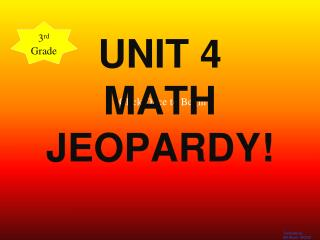DownloadDownload PresentationUNIT 4 MATH JEOPARDY!

# UNIT 4 MATH JEOPARDY!

Télécharger la présentation## UNIT 4 MATH JEOPARDY!

- - - - - - - - - - - - - - - - - - - - - - - - - - - E N D - - - - - - - - - - - - - - - - - - - - - - - - - - -
##### Presentation Transcript

1. 3rd Grade UNIT 4 MATHJEOPARDY! Click Once to Begin

2. JEOPARDY! Fact Families Big Numbers Laws of Multiplication Multiplication Area of Rectangles Multiplication Number Stories 100 100 100 100 100 100 200 200 200 200 200 200 300 300 300 300 300 300 400 400 400 400 400 400 500 500 500 500 500 500

3. Fact Families 100 Complete the Fact Family 3 x 5 =______ 5 x 3 =______ __ ÷ 3 = 5 __ ÷ 5 = 3 Correct! 3 x 5 =15 5 x 3 =15 15÷ 3 = 5 15 ÷ 5 = 3

4. Fact Families 200Complete the fact families. 6 x 5 =______ 5 x 6 =______ __ ÷ 5 = 6 __ ÷ 6 = 5 Correct 6 x 5 = 30 5 x 6 = 30 30 ÷ 5 = 6 30 ÷ 6 = 5

5. Fact Families 300Complete the fact families. 7 x 6 =______ 6 x 7 =______ __ ÷ 6 = 7 __ ÷ 7 = 6 CORRECT! 7 x 6 = 42 6 x 7 = 42 42÷ 6 = 7 42÷ 7 = 6

6. Fact Families 400Complete the fact families. 8 x 4 =______ 4 x 8 =______ __ ÷ 4 = 8 __ ÷ 8 = 4 CORRECT! 8 x 4 = 32 4 x 8 = 32 32 ÷ 4 = 8 32 ÷ 8 = 4

7. Fact Families 500Complete the fact families. 9 x 8 =______ 8 x 9 =______ __ ÷ 9 = 8 __ ÷ 8 = 9 CORRECT! 9 x 8 = 72 8 x 9 72 72 ÷ 8 = 9 72 ÷ 9 = 8

8. Write a number that is: 100 more than 405 Big Numbers 100 CORRECT! 505

9. Write a number that is: 100 more than 1,543 Big Numbers 200 Correct! 1,643

10. Big Numbers 500Write a number that is:100 less than 10,309 Correct! 10,209

11. Fill in the blank use >, <, or =. 3 x 4 ____ 4 x 3 Laws of Multiplication 100 Correct! 3 x 4 = 4 x 3

12. Fill in the blank use >, <, or =. 0 x 4 ____ 2 x 2 Laws of Multiplication 200 Correct! 0 x 4 < 2 x 2

13. Daily Double!!! Fill in the blank use >, <, or =. 9 x 4 ____ 10 x 2 Laws of Multiplication 300 Correct! 9 x 4 > 10 x 2

14. Laws of Multiplication 400 Fill in the blank use >, <, or =.7 x 7 ____ 6 x 9 Correct! 7 x 7 < 6 x 9

15. Laws of Multiplication 500 Fill in the blank use >, <, or =.12 x 12 ____ 72 x 2 Correct! = 12 x 12 = 72 x 2

16. Fill In the blanks Multiplication 100 Correct! 6 15 3 10 your choice

17. Fill In the blanks. Multiplication 200 Rule: x3 Correct! 24; 18; 12; 6; 0; your choice

18. Multiplication 300Fill in the blanks Rule: x4 Correct! 36; 28; 20; 12; 0; your choice

19. Fill in the blanks. Multiplication 400 Correct! 3; 5; 21; 30; your choice

20. Multiplication 500Fill in the blanks. Rule: x6 Correct! 54, 36, 30, 12, 0, your choice

21. Find the area of the rectangle. Area of a Rectangle100 CORRECT! Area = 12 square units

22. Area of a Rectangle 200 Correct! 2 x 4 = 8 Area: 8 square units

23. Area of a Rectangle 300Find the area of the rectangle. Correct! 21 square cm

24. Area of a Rectangle 400 Correct!

25. Area of a Rectangle 500 CORRECT!

26. Multiplication Number Stories 100 Correct! 3 x 6 = 18

27. Multiplication Number Stories 200 Correct! 5 x 6 = 30 5 teams

28. Multiplication Number Stories 300 Correct! 3 x 12 = 36 36 pencils

29. Number Model:_______________ Multiplication Number Stories 400 Correct! 4 x 10 = 40 40 stock cars

30. Number Model:_________________ Multiplication Number Stories 500 Correct! 20 cards 5 x 20 = 100

31. FINAL JEOPARDY Mrs. Jones told her class that they could calcuate the difference between 20 x 2 and 20 x 3 without doing any multiplication. Show how Mrs. Jones’ class might have solved the problem. You can use pictures, arrays, number models, diagrams, coins or counters.

32. Final Jeopardy 20 x 2 and 30 x 2 20 + 20 = 40 20 + 20 + 20 = 60 60 – 40 = 20 I know that multiplication is repeated addition so 20 x 2 is the same as 20 + 20 and 20 x 3 is the same as 20 + 20 + 20. 60 – 40 = 20, so the difference is 20!

33. Thank you for playing JEOPARDY!